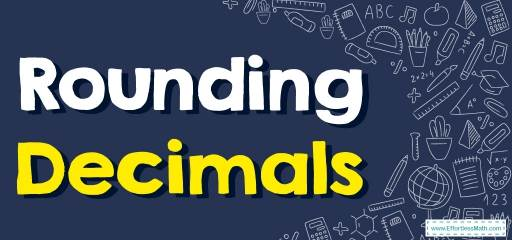# How to Round Decimals? (+FREE Worksheet!)

Want an explanation and example of how to round decimal numbers? Join us to learn and practice how to round decimals in a few simple steps.Fractions whose denominator is $$10$$ or $$100$$ or $$1000$$ or … are called decimal fractions.

Note: In decimal numbers, the first digit after the decimal point is called the tenth, the second digit is the hundredth, the third digit is the thousandth, and so on.

## Step by step guide to rounding decimals

• Numbers are the key to accessing calculations. But working with decimal numbers is more difficult than with integers. In particular, our minds are not prepared enough to work on decimal numbers, and it is easier to do calculations, even approximately, with the help of integers. To do this, before performing the calculations, we “round” the decimal numbers to quickly increase our calculations. Of course, by doing so, we have reduced the accuracy of the calculations. When we round a number, the value of the result is an integer that is very close to the original number and can be an alternative to calculations. In this way, results are easier to understand when exact values are not too important.
• ُStep 1: First, you’ll need to remember your place values: For example:
$$12.4568$$
$$1$$:tens, $$2$$: ones, $$4$$: tenths,
$$5$$: hundredths, $$6$$: thousandths, $$8$$: tens thousandths
• Step 2: To round a decimal, find the place value you’ll round to.
• Step 3: Find the digit to the right of the place value you’re rounding to. If it is $$5$$ or bigger, add $$1$$ to the place value you’re rounding to and remove all digits on its right side. If the digit to the right of the place value is less than $$5$$, keep the place value and remove all digits on the right.

### Rounding Decimals – Example 1:

Round $$1.9287$$ to the thousandth place value.

Solution:

First look at the next place value to the right, (tens thousandths). It’s $$7$$ and it is greater than $$5$$. Thus add $$1$$ to the digit in the thousandth place.
The thousandth place is $$8$$. $$→ 8 \ + \ 1=9$$, then, the answer is $$1.929$$

### Rounding Decimals – Example 2:

Round $$9.4126$$ to the nearest hundredth.

Solution:

First, look at the next place value to the right of the hundredth place. It’s $$2$$ and it is less than $$5$$, thus removing all the digits to the right. Then, the answer is $$9.41$$.

### Rounding Decimals – Example 3:

Round $$2.1837$$ to the tenth place value.

Solution:

First look at the next place value to the right, (hundredth). It’s $$8$$ and it is greater than $$5$$. Thus add $$1$$ to the digit in the tenth place.
Tenth place is $$1$$.
$$→ 1+1=2$$, then, the answer is $$2.2$$

### Rounding Decimals – Example 4:

$$2.1837$$ rounded to the nearest hundredth.

Solution :

First, look at the next place value to the right of the hundredth. It’s $$3$$ and it is less than $$5$$, thus removing all the digits to the right. Then, the answer is $$2.18$$.

## Exercises for Rounding Decimals

### Round each decimal number to the nearest place indicated.

1. $$\color{blue}{0.\underline{2}3}$$
2. $$\color{blue}{4.\underline{0}4}$$
3. $$\color{blue}{5.\underline{6}23}$$
4. $$\color{blue}{0.\underline{2}66}$$
5. $$\color{blue}{116.\underline{5}14}$$
6. $$\color{blue}{8.\underline{0}6}$$

1. $$\color{blue}{0.2}$$
2. $$\color{blue}{4 }$$
3. $$\color{blue}{5.6}$$
4. $$\color{blue}{0.3}$$
5. $$\color{blue}{116.5}$$
6. $$\color{blue}{8.1}$$

### What people say about "How to Round Decimals? (+FREE Worksheet!) - Effortless Math: We Help Students Learn to LOVE Mathematics"?

No one replied yet.

X
30% OFF

Limited time only!

Save Over 30%

SAVE $5 It was$16.99 now it is \$11.99